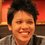# Prove (or disprove): Let $f_n[g(x)]$ denote the $n^\text{th}$ derivative of $g(x)$ at $x=1$, then $f_n[^n(x)]=f_n[^{n+1}(x)]=f_n[^{n+2}(x)]=\ldots$ for all positive integers $n$.

https://brilliant.org/problems/separation-at-tinfty/

Hey Abhishek, we think your problem is great for the following reason:

• Astonishing result: It is interesting to find that even after having equal top speeds, Tom will never be able to catch Jerry.

We have made the following key improvement to your problem that makes it even better:

• Imagery: Added a skin to the problem. Adding the story of Tom chasing Jerry makes the problem setup more interesting and engaging.Note by Pi Han Goh
5 years, 6 months ago

This discussion board is a place to discuss our Daily Challenges and the math and science related to those challenges. Explanations are more than just a solution — they should explain the steps and thinking strategies that you used to obtain the solution. Comments should further the discussion of math and science.

When posting on Brilliant:

• Use the emojis to react to an explanation, whether you're congratulating a job well done , or just really confused .
• Ask specific questions about the challenge or the steps in somebody's explanation. Well-posed questions can add a lot to the discussion, but posting "I don't understand!" doesn't help anyone.
• Try to contribute something new to the discussion, whether it is an extension, generalization or other idea related to the challenge.

MarkdownAppears as
*italics* or _italics_ italics
**bold** or __bold__ bold
- bulleted- list
• bulleted
• list
1. numbered2. list
1. numbered
2. list
Note: you must add a full line of space before and after lists for them to show up correctly
paragraph 1paragraph 2

paragraph 1

paragraph 2

[example link](https://brilliant.org)example link
> This is a quote
This is a quote
    # I indented these lines
# 4 spaces, and now they show
# up as a code block.

print "hello world"
# I indented these lines
# 4 spaces, and now they show
# up as a code block.

print "hello world"
MathAppears as
Remember to wrap math in $$ ... $$ or $ ... $ to ensure proper formatting.
2 \times 3 $2 \times 3$
2^{34} $2^{34}$
a_{i-1} $a_{i-1}$
\frac{2}{3} $\frac{2}{3}$
\sqrt{2} $\sqrt{2}$
\sum_{i=1}^3 $\sum_{i=1}^3$
\sin \theta $\sin \theta$
\boxed{123} $\boxed{123}$

Sort by:

@Pi Han Goh so it would be fine right?? If I do the same.

- 5 years, 3 months ago

- 5 years, 6 months ago

Yesterday, you suspected that I had a "trick" up my sleeve... well, that was my trick (in the case $n=3$) ;)

- 5 years, 6 months ago

haha! I thought you had ANOTHER trick up your sleeves! I was persistent in solving it my way but it's over 4 pages long and the equations are enormous so I gave up half way through.

- 5 years, 6 months ago

Great observation! It's easy to see why this would be true. Let $T_n(f(x))$ be the Taylor polynomial of $f(x)$ at $x=0$.

If $T_n(f(x))=T_n(g(x))$ for two functions, then $T_{n+1}(\ln(x+1)f(x))=T_{n+1}(\ln(x+1)g(x))$; since $\ln(x+1)$ does not have a constant term, the degree gets "pushed up". This in turn implies that $T_{n+1}((x+1)^{f(x)})=T_{n+1}((x+1)^{g(x)})$, by exponentiation.

Now $T_1(x+1)=T_1\left((x+1)^{x+1}\right)$, by inspection ; applying the above observation $n-1$ times, we find that $T_n\left(^n(x+1)\right)=T_{n}\left(^{n+1}(x+1)\right)$ and therefore $T_n\left(^n(x+1)\right)=T_{n}\left(^m(x+1)\right)$ for all $m\geq{n}$ .

Let $D_n(f(x))$ be the nth derivative of $f(x)$ at $x=0$. Since $D_n$ is determined by $T_n$, we have $D_n\left(^n(x+1)\right)=D_{n}\left(^m(x+1)\right)$ for all $m\geq{n}$ .

In particular, $D_3\left(^7(x+1)\right)=D_{3}\left(^3(x+1)\right)=9$, as we saw in Pi Han Goh's earlier problem.

- 5 years, 6 months ago

You take the words out of my mouth. Haha, just kidding. Nice! I didn't thought it was that simple. THANKYOU

- 5 years, 6 months ago

If we do it for $n=3$ only, its pretty simple.

Using our earlier work, the only "new" computation you need is $T_3\left(\ln(x+1)(x+1)^{(x+1)^{x+1}}\right)=x+\frac{x^2}{2}+\frac{5x^3}{6}$ . You realize that this is the same as $T_3\left(\ln(x+1)(x+1)^{x+1}\right)$ ... done!

Thank you so much for the fruitful cooperation! I will use these kinds of problems in the Final Exam in Calculus at Harvard this summer... I will let you know about the results ;)

- 5 years, 6 months ago